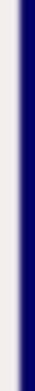homework help forum today's math problem links utilities previous math problem feedback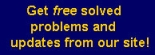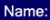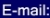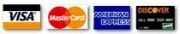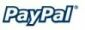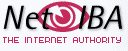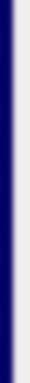# MATH HOMEWORK HELP

14. Using implicit differentiation, find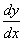if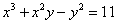. Also evaluate the derivative at x =2 and y = 3

Solution: Differentiating the equationyields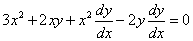Solving forwe obtain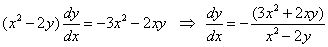Replacing at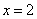and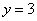leads to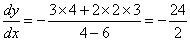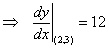MGT - Your source of Math and Statistics Homework Help